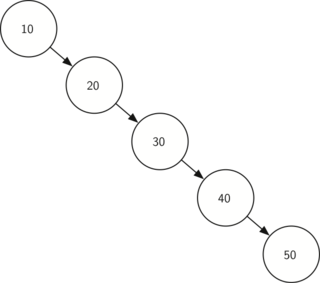# 8.13. Search Tree Analysis¶

With the implementation of a binary search tree now complete, we will do a quick analysis of the methods we have implemented. Let’s first look at the put method. The limiting factor on its performance is the height of the binary tree. Recall from the vocabulary section that the height of a tree is the number of edges between the root and the deepest leaf node. The height is the limiting factor because when we are searching for the appropriate place to insert a node into the tree, we will need to do at most one comparison at each level of the tree.

What is the height of a binary tree likely to be? The answer to this question depends on how the keys are added to the tree. If the keys are added in a random order, the height of the tree is going to be around $$\log_2{n}$$ where $$n$$ is the number of nodes in the tree. This is because if the keys are randomly distributed, about half of them will be less than the root and half will be greater than the root. Remember that in a binary tree there is one node at the root, two nodes in the next level, and four at the next. The number of nodes at any particular level is $$2^d$$ where $$d$$ is the depth of the level. The total number of nodes in a perfectly balanced binary tree is $$2^{h+1}-1$$, where $$h$$ represents the height of the tree.

A perfectly balanced tree has the same number of nodes in the left subtree as the right subtree. In a balanced binary tree, the worst-case performance of put is $$O(\log_2{n})$$, where $$n$$ is the number of nodes in the tree. Notice that this is the inverse relationship to the calculation in the previous paragraph. So $$\log_2{n}$$ gives us the height of the tree, and represents the maximum number of comparisons that put will need to do as it searches for the proper place to insert a new node.

Unfortunately it is possible to construct a search tree that has height $$n$$ simply by inserting the keys in sorted order! An example of such a tree is shown in Figure 6. In this case the performance of the put method is $$O(n)$$.Figure 6: A skewed binary search tree would give poor performance

Now that you understand that the performance of the put method is limited by the height of the tree, you can probably guess that other methods, get, in, and del, are limited as well. Since get searches the tree to find the key, in the worst case the tree is searched all the way to the bottom and no key is found. At first glance del might seem more complicated, since it may need to search for the successor before the deletion operation can complete. But remember that the worst-case scenario to find the successor is also just the height of the tree which means that you would simply double the work. Since doubling is a constant factor it does not change worst case

Q-1: The worst-case performance of the del function is O()?